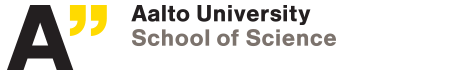Department of Mathematics and Systems Analysis

# Research reports by Wolfgang Desch

Here are the reports authored by Wolfgang Desch. See the reports of all authors.

## Aalto University publication series Science + Technology

ST 1/2011
Maximal Regularity for Stochastic Integral Equations   [pdf]
Received 2011-01-07 * stochastic integral equations, maximal regularity, bounded H-infinity calculus, Volterra equations, singular kernels * AMS 60H15, 60H20, 45N05 * 15 pages

## Helsinki University of Technology Institute of Mathematics Research Reports A

A583
Semilinear stochastic integral equations in Lp   [pdf][ps]
Received 2009-12-31 * semilinear stochastic integral equations, stochastic fractional differential equation, regularity, nonnegative operator, Volterra equation, singular kernel * AMS 60H15, 60H20, 45N05 * 26 pages
A581
An Lp-theory for stochastic integral equations   [pdf][ps]
Received 2009-11-11 * stochastic integral equations, stochastic fractional differential equation, regularity, nonnegative operator, Volterra equation, singular kernel * AMS 60H15, 60H20, 45N05 * 25 pages
A547
A generalization of an inequality by N. V. Krylov   [pdf][ps]
Received 2008-06-05 * Littlewood-Paley inequality, heat kernel, resolvent operators, stochastic integral equations * AMS 60H15, 45K05 * 34 pages
A513
On a stochastic parabolic integral equation   [pdf][ps] abstract: [pdf][ps]
Received 2006-10-26 * Stochastic integral equations, Krylovs method * AMS 60H20 45R05 * 12 pages

Page content by: webmaster-math [at] list [dot] aalto [dot] fi